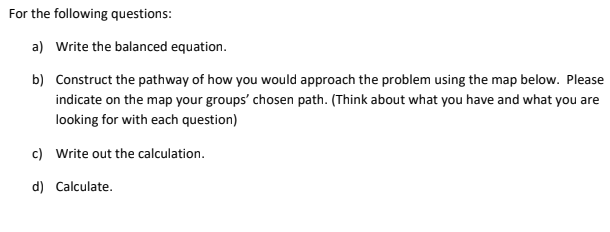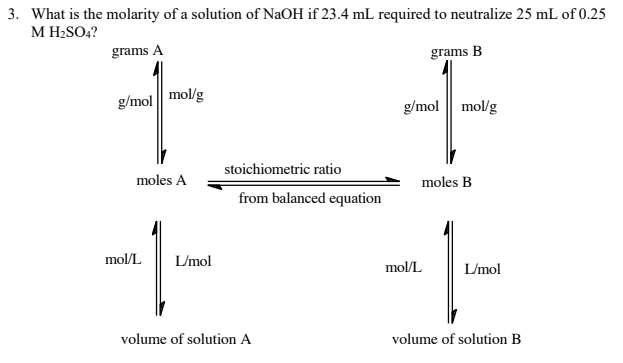# For the following questions: a) Write the balanced equation b) Construct the pathway of how you would approach the problem using the map below. Please indicate on the map your groups' chosen path. (Think about what you have and what you are looking for with each question) c) Write out the calculation. d) Calculate. 3. What is the molarity of a solution of NaOH if 23.4 mL required to neutralize 25 ml of 0.25 M H2SO4?